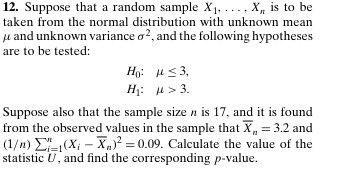# Question 12. Suppose that a random sample $$X_{1}, \ldots, X_{n}$$ is to be taken from the normal distribution with unknown mean $$\mu$$ and unknown variance $$\sigma^{2}$$, and the following hypotheses are to be tested:$\begin{array}{ll}H_{0}: & \mu \leq 3, \\H_{1}: & \mu>3 .\end{array}$Suppose also that the sample size $$n$$ is 17 , and it is found from the observed values in the sample that $$\bar{X}_{n}=3.2$$ and $$(1 / n) \sum_{i=1}^{n}\left(X_{i}-\bar{X}_{n}\right)^{2}=0.09$$. Calculate the value of the statistic $$U$$, and find the corresponding $$p$$-value.LPSSXJ The Asker · Probability and Statistics
12. Suppose that a random sample $$X_{1}, \ldots, X_{n}$$ is to be taken from the normal distribution with unknown mean $$\mu$$ and unknown variance $$\sigma^{2}$$, and the following hypotheses are to be tested:
$\begin{array}{ll} H_{0}: & \mu \leq 3, \\ H_{1}: & \mu>3 . \end{array}$
Suppose also that the sample size $$n$$ is 17 , and it is found from the observed values in the sample that $$\bar{X}_{n}=3.2$$ and $$(1 / n) \sum_{i=1}^{n}\left(X_{i}-\bar{X}_{n}\right)^{2}=0.09$$. Calculate the value of the statistic $$U$$, and find the corresponding $$p$$-value.Transcribed Image Text: 12. Suppose that a random sample $$X_{1}, \ldots, X_{n}$$ is to be taken from the normal distribution with unknown mean $$\mu$$ and unknown variance $$\sigma^{2}$$, and the following hypotheses are to be tested: $\begin{array}{ll} H_{0}: & \mu \leq 3, \\ H_{1}: & \mu>3 . \end{array}$ Suppose also that the sample size $$n$$ is 17 , and it is found from the observed values in the sample that $$\bar{X}_{n}=3.2$$ and $$(1 / n) \sum_{i=1}^{n}\left(X_{i}-\bar{X}_{n}\right)^{2}=0.09$$. Calculate the value of the statistic $$U$$, and find the corresponding $$p$$-value.
More
Transcribed Image Text: 12. Suppose that a random sample $$X_{1}, \ldots, X_{n}$$ is to be taken from the normal distribution with unknown mean $$\mu$$ and unknown variance $$\sigma^{2}$$, and the following hypotheses are to be tested: $\begin{array}{ll} H_{0}: & \mu \leq 3, \\ H_{1}: & \mu>3 . \end{array}$ Suppose also that the sample size $$n$$ is 17 , and it is found from the observed values in the sample that $$\bar{X}_{n}=3.2$$ and $$(1 / n) \sum_{i=1}^{n}\left(X_{i}-\bar{X}_{n}\right)^{2}=0.09$$. Calculate the value of the statistic $$U$$, and find the corresponding $$p$$-value.